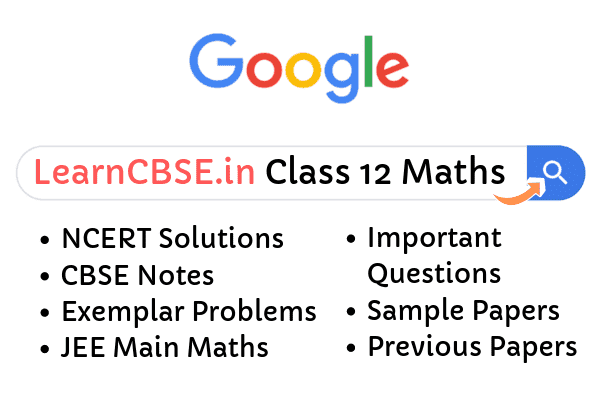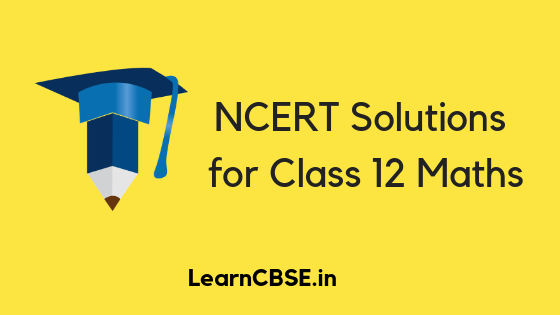NCERT Solutions for Class 12 Maths has been written to help students like you who are appearing for different board exams as well as the JEE. It provides sound knowledge and understanding of all important concepts covered in each chapter in the Class 12 Maths NCERT textbook. There are 13 important chapters from the NCERT textbook. Both problems and their detailed solutions are provided. The solutions have been devised by India’s best teachers to help students grasp basic concepts better and faster.

## NCERT Solutions for Class 12 Maths All Chapters

NCERT Maths Class 12 Solutions are given below for all chapter. Select chapter number to view Class 12 Maths NCERT chapter wise solutions.

If you are looking for Class 12 Maths NCERT Solutions, there is no better place to be than right here. We provide Maths NCERT solutions class 12 that are precise, to the point and absolutely error free. With NCERT reputed to be the best textbooks for school education, we make the solutions that are at par with the excellent textbooks.

### Important Questions for Class 12 Maths Chapter wise

Relations and Functions

Inverse Trigonometric Functions

Matrices

Determinants

Continuity and Differentiability

Application of Derivatives

Integrals

Differential Equation

Vector Algebra

Three Dimensional Geometry

Linear Programming

Probability

### NCERT Exemplar Class 12 Maths

RD Sharma Class 12 Maths Solutions

### Class 12 Maths NCERT Solutions

Class 12 Maths is key in the preparation for boards as well as JEE Main and Advance, and our solutions are made keeping in mind the very same thing. The solutions are concise, and also discuss alternative methods to solve some problems. We make it a point to provide the students the best help they can get, and the solutions reflect just that.

The National Council of Educational Research and Training (NCERT) is an autonomous organization of the Government of India that was established in 1961 as a literary, scientific and charitable society under the Societies’ Registration Act (Act XXI of 1860). Its headquarters are located at Sri Aurobindo Marg in New Delhi. It sets the curriculum for all schools that follow the Central Board of Secondary Education (CBSE) across the country. Class 12 Maths NCERT Solutions is the perfect guide to help you prepare for CBSE exams as well as engineering entrances like the JEE.

Your performance in the board exams will play a role in determining your admission into a good college or even your dream college! That will undoubtedly induce a lot of pressure upon you and you may tend to get demotivated or stressed out. To help you overcome such situations, LearnCBSE is a platform all the way helps you to crack your target. if you have any queries leave in the comments box.### NCERT Solutions for Class 12 Maths Chapter 1 Relations and Functions

The chapter builds up on the concepts of relations, functions, domain and codomain introduced in Class 11. The students explore several real valued functions, and their graphs. The chapter comprises of four exercises that consolidate the concepts explained in the chapter.

### NCERT Solutions for Class 12 Maths Chapter 2 Inverse Trigonometric Functions

This chapter plays in the restricted range of trigonometric functions that enables them to be one on one, and hence makes inverse of the functions visible. This chapter explores the inverse trigonometric through two exercises that teach the students the behavior of the functions, their graphical representations, and the elementary properties. These prove to be of more value in integration later.

### NCERT Solutions for Class 12 Maths Chapter 3 Matrix

This chapter takes you through the properties of one of the most important tools of Mathematics, namely Matrices. The students learn the basics of Matrices and Matrix Algebra..

### NCERT Solutions for Class 12 Maths Chapter 4 Determinants

Correctly preceded by Matrix, this chapter deals with an important part of Matrices, namely Determinants. The chapter deals with determinants of orders upto 3, and their cofactors and inverses.This chapter also talks about the applications of determinants, like calculation of area of triangles, adjoint of matrices, consistency of a set of linear equations.

### NCERT Solutions for Class 12 Maths Chapter 5 Continuity and Differentiability

This chapter picks up after the Differentiation of Functions introduced in Class 11. The chapter explores the important concepts of Continuity, Differentiability and establishes the relationship between them. It also deals with the continuity and differentiability of Inverse Trigonometric Functions. It also introduces exponential and logarithmic functions.

### NCERT Solutions for Class 12 Maths Chapter 6 Application of Derivatives

With Continuity and Differentiability marking the start of Calculus, this chapter deals with the differentiability of functions, and introduction to derivatives. It delves into the derivatives of specific functions, and their applications, namely the tangents and normal to curves, and the rate of change in some quantities. It also talks about increasing and decreasing functions.

### NCERT Solutions for Class 12 Maths Chapter 7 Integrals

This is one of the most important and time consuming chapters in the whole Class 12 Mathematics. This chapter deals with integration of functions, and its applications in terms of calculation of areas. This marks the introduction to Integral Calculus. There are a total of 11 exercises in this chapter, making it a very practice driven part of the syllabus.

### NCERT Solutions for Class 12 Maths Chapter 8 Application of Integrals

This chapter deals with the applications of integrals in calculating areas under simple curve, lines, parabolas and ellipses. The two exercises provide enough questions for practicing the area calculations.

### NCERT Solutions for Class 12 Maths Chapter 9 Differential Equations

This chapter introduces the concept of Differential equations, the general and particular solutions, and the order and degree of an equation. This also talks about the applications of differential equations in the six exercises.

### NCERT Solutions for Class 12 Maths Chapter 10 Vector Equations

This chapter deals with the vector quantities, that is, the quantities with both magnitude and direction. The Vector algebra summarises the rules of addition of vector quantities, and other important properties. Vector Algebra plays a big role in Physics.

### Class 12 Maths Chapter 11 Three Dimensional Geometry

This chapter utilises vectors to calculate the distance between lines, a point and a line, and a line and a plane, along with the angles between them.It also introduces the concept of direction cosines

### Class 12 Maths Chapter 12 Linear Programming

This chapter explores the concept of linear programming problems, and their solutions using graphical method only.

### Class 12 Maths Probability

This chapter deals with the concept of Conditional Probability and the encounters of the same in our daily lives. This explores Bayes’ Theorem, the multiplication rule, and the independence of events. This chapter is best understood by practicing as many questions as possible.

#### NCERT Solution

We hope you will have great learning experience while using the solutions. You can download the solutions by clicking on the links above in the description.